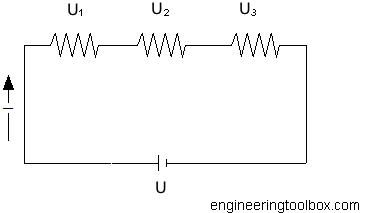Engineering ToolBox - Resources, Tools and Basic Information for Engineering and Design of Technical Applications!

# Electrical Series Circuits

## Voltage and current in electrical series circuits.

A series circuit has only one path for the electrons to flow.

The Series Circuit Rules:

1. The total current in the circuit is equal to the current in any other part of the circuit.
2. The total voltage in a series circuit is equal to the sum of the voltages across all parts in the circuit.
3. The total resistance in a series circuit is equal to the resistances of all parts in the circuit.In series circuits the sum of voltages is equal to the applied voltage and can be expressed as

U = U1 + U2 + ... + Un                  (1)

where

U = applied voltage from the battery or source (volts, V)

U1..n = voltage over each resistor (volts, V)

From Ohms Law

Un = In Rn                     (2)

where

In = current (amps, A)

Rn = resistance (ohms, Ω)

According rule 1 the currents in a series circuit are equal. This can be expressed as

I1 = I2 = .. = In                    (3)

- equation (1) can be modified to

R = R1 + R2 + .. + Rn                 (4)

where

R = total circuit resistance in the circuit (ohms, Ω)

### Example - Series Circuit

Three resistors - 1.25 Ω, 0.5 Ω and 1.5 Ω - are connected in series to a 12 V car battery. The total resistance in the circuit can be calculated as

R = (1.0 Ω) + (0.5 Ω) + (1.5 Ω)

= 3 Ω

The current in the series circuit can be calculated as

I = U / R

= 12 / 3

= 4 amps

The power dissipated in the circuit can be calculated

P = R I2

= (3 Ω) (4 amps)2

= 48 watts

## Related Topics

• Electrical - Electrical units, amps and electrical wiring, wire gauge and AWG, electrical formulas and motors.

## Engineering ToolBox - SketchUp Extension - Online 3D modeling!

Add standard and customized parametric components - like flange beams, lumbers, piping, stairs and more - to your Sketchup model with the Engineering ToolBox - SketchUp Extension - enabled for use with the amazing, fun and free SketchUp Make and SketchUp Pro .Add the Engineering ToolBox extension to your SketchUp from the SketchUp Pro Sketchup Extension Warehouse!

Translate

## Privacy

We don't collect information from our users. Only emails and answers are saved in our archive. Cookies are only used in the browser to improve user experience.

Some of our calculators and applications let you save application data to your local computer. These applications will - due to browser restrictions - send data between your browser and our server. We don't save this data.

## Citation

• Engineering ToolBox, (2008). Electrical Series Circuits. [online] Available at: https://www.engineeringtoolbox.com/series-circuits-d_1383.html [Accessed Day Mo. Year].

Modify access date.

. .

#### Scientific Online Calculator3 30